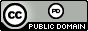시간 제한 메모리 제한 제출 정답 맞은 사람 정답 비율
5 초 1024 MB 0 0 0 0.000%

## 문제

The Computer Science Library on campus needs to be temporarily closed for renovations and you have been put in charge of the storage of all the library's books.  After getting over the shock of being selected as well as the shock that the campus actually had a CS library, you get down to work.  The books have already been packed in identical boxes which all have the same weight.  You want to stack these boxes on wooden pallets in case the storage room gets flooded, but you have a small problem: you don't know how many boxes you can stack before the pallets collapse under the weight.  We'll call the maximum number of boxes that can rest on a pallet the box limit.

You could methodically place one box on a pallet, then a second, a third, etc., until the pallet breaks but that seems very time consuming (and leads to a very boring contest problem).  But if you have one or more pallets to experiment with you might be able to determine the box limit quicker.  For example, suppose because of the size of the boxes and the height of the storage room ceiling the maximum number of boxes in any stack is $3$.  With just one pallet you could first try one box.  If the pallet collapses, well, you need to go out and get some stronger pallets.  If the pallet holds then you could try a second box. If the pallet collapses now you know the box limit is $1$; otherwise, you try a third box and that will let you know whether the box limit is $2$ (if the pallet collapses) or $3$ (if the pallet doesn't collapse).  This approach requires at most three different experiments.  However, if you had two pallets you could determine the box limit with at most only two experiments: first you try two boxes on the first pallet; if it holds, you try a third which will let you know whether the box limit is $2$ or $3$.  If the first pallet collapses in the first experiment, you haul out the second pallet and place a single box on it.  The result of that experiment tells you if the box limit is $1$ or $0$.

You are not exactly sure about the height of the storage room (which dictates the maximum possible boxes that could be stacked) or how many pallets you have at your disposal to experiment with.  What you would like to know is when given this information, what is the minimum worst-case number of experiments you need to run given an optimal strategy.  Too bad you didn't know about the CS Library earlier -- maybe there was something in one of the books that would help you now.

## 입력

Input consists of a single line containing two positive integers $n$ and $m$ ($n \leq 5\,000, m \leq 20$), where $n$ indicates the maximum number of boxes that can be stacked (regardless of whether a pallet would be collapsed by them or not) and $m$ is the number of available pallets to experiment with.

## 출력

Output the minimum worst-case number of experiments that the optimal strategy would require followed by the number of boxes to use in the first experiment.  If there is a range of boxes that can be used in the first experiment display the minimum and maximum values of this range separated by a hyphen; if there is only one such number simply output that number.

## 예제 입력 1

3 1


## 예제 출력 1

3 1


## 예제 입력 2

3 2


## 예제 출력 2

2 2


## 예제 입력 3

4 2


## 예제 출력 3

3 1-3


## 출처• 문제를 만든 사람: Bob Roos